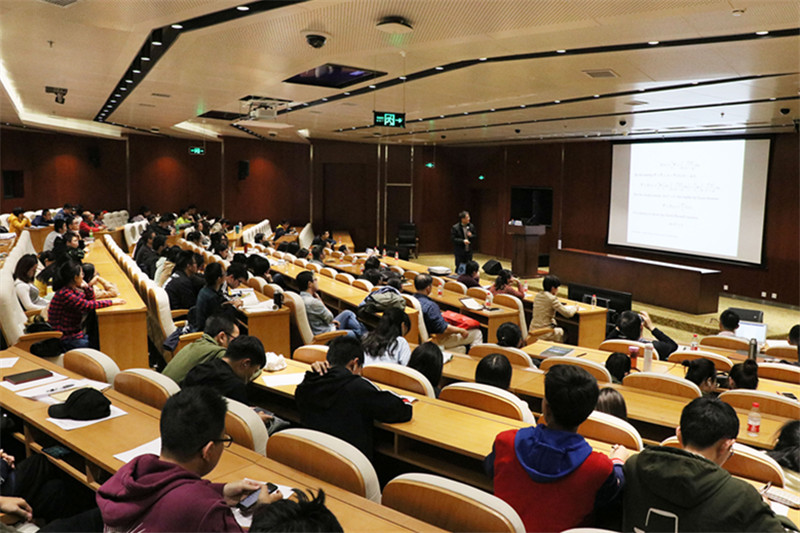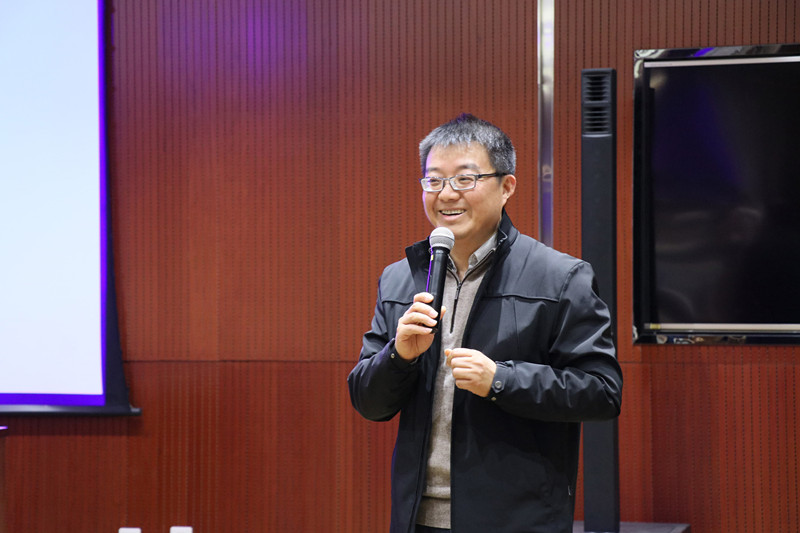• FeedBack
• Search

# News

## Chen Zhiming Elaborated Mathematical Methods in Computational Electromagnetism

On November 9, 2018, Chen Zhiming, Academician of CAS and researcher of Academy of Mathematics and Systems Science, Chinese Academy of Sciences gave a lecture entitled “Mathematical Methods in Computational Electromagnetism” in Peiyang Maths Forum. The lecture was held in the lecture hall of the administrative building of Peiyang Park Campus and was presided by Sun Xiaotao, the dean of School of Mathematics.

Chen Zhiming started from Coulomb's law, passes through the divergence theorem, and went to Maxwell equations. The computational electromagnetism began with Maxwell equations. Through the electromagnetic field modeling, he described the development and recent status of some mathematical methods in the computation.Professor Chen firstly introduced the establishment and the development of the finite element method. He mentioned that the finite element method originated from Galerkin method and pointed out that the finite element method is an important method in mathematics, which had been widely used. The a priori estimate reveals the shortcoming of the traditional finite element method in convergence speed, so that the posteriori error estimates and adaptive finite element method gradually embarked on historical stage.Next, Professor Chen illustrated how mathematics played an important role in solving real problems by the examples of eddy current and the electromagnetic field computation.

Finally, Professor Chen concluded that the solution of mathematical model is not equal to the solution in practical problems. He expounded that the uniqueness of the solution is very important in mathematical thinking. He pointed out that scientific and engineering computation is the third method to carry out scientific research.

A freshmen questioned that if a model dose not match the fact, where dose the error come from and from which aspects should it be perfected? Professor Chen answered that it might be caused by a wrong model or by a wrong computation. For the first case, we need to verify the model through experiments. For the second case, we shall first ensure the correctness of algorithm. Another student questioned that whether AI would exist without computational mathematics. Professor Chen responded with a smile that there was no clear and definite link between computational mathematics and AI. Mathematical research concentrates on theoretical issues while AI pays more attention to practical issues. Software is the pillar of AI, and the core of software is algorithm. It also requires us to devote a lot of energy and efforts to breaking through various development constraints. Professor Chen believed that the scientists have been working hard to seek the truth.

By: Zhang Jiawei, Ma Changying

Editors: Qin Mian and Keith Harrington